# Analyzing US baby names over the years

TidyTuesday 2022 Week 12: Baby names.

R
TidyTuesday
data visualization
R setup
``````library(tidyverse)
library(tidytuesdayR)
library(gt)
library(rmarkdown)
library(patchwork)
library(ggtext)
library(glue)

library(dunnr)
extrafont::loadfonts(device = "win", quiet = TRUE)
theme_set(theme_td_minimal())
set_geom_fonts()
set_palette()

sex_pal <- c("M" = "#8ecefd", "F" = "#f88b9d")``````

``tt <- tidytuesdayR::tt_load("2022-03-22")``
``--- Compiling #TidyTuesday Information for 2022-03-22 ----``
``--- There are 8 files available ---``
``--- Starting Download ---``
``````
``--- Download complete ---``

There are 8 data frames this week. For this post, I’ll be exploring `babynames`, which are baby name counts by `year` and `sex` in the US:

``````babynames <- tt\$babynames
glimpse(babynames)``````
``````Rows: 1,924,665
Columns: 5
\$ year <dbl> 1880, 1880, 1880, 1880, 1880, 1880, 1880, 1880, 1880, 1880, 1880,…
\$ sex  <chr> "F", "F", "F", "F", "F", "F", "F", "F", "F", "F", "F", "F", "F", …
\$ name <chr> "Mary", "Anna", "Emma", "Elizabeth", "Minnie", "Margaret", "Ida",…
\$ n    <dbl> 7065, 2604, 2003, 1939, 1746, 1578, 1472, 1414, 1320, 1288, 1258,…
\$ prop <dbl> 0.07238359, 0.02667896, 0.02052149, 0.01986579, 0.01788843, 0.016…``````

## Data exploration

The data span the following years:

``c(min(babynames\$year), max(babynames\$year))``
`` 1880 2017``

The `prop` variable is presumably the proportion of babies with that `name` and `sex` in a given year:

``````babynames %>%
group_by(sex, year) %>%
mutate(n_total = sum(n)) %>%
ungroup() %>%
mutate(prop_manual = n / n_total) %>%
select(prop, prop_manual)``````
``````# A tibble: 1,924,665 × 2
prop prop_manual
<dbl>       <dbl>
1 0.0724      0.0776
2 0.0267      0.0286
3 0.0205      0.0220
4 0.0199      0.0213
5 0.0179      0.0192
6 0.0162      0.0173
7 0.0151      0.0162
8 0.0145      0.0155
9 0.0135      0.0145
10 0.0132      0.0142
# … with 1,924,655 more rows
# ℹ Use `print(n = ...)` to see more rows``````

Not quite – my manually calculated `prop_manual` is slightly higher. It may be due to un-documented names not being counted in the `babynames` data frame. The `applicants` data has the number of social security number applications. Is this the denominator?

``````babynames %>%
left_join(tt\$applicants, by = c("year", "sex")) %>%
mutate(prop_manual = n / n_all) %>%
select(prop, prop_manual)``````
``````# A tibble: 1,924,665 × 2
prop prop_manual
<dbl>       <dbl>
1 0.0724      0.0724
2 0.0267      0.0267
3 0.0205      0.0205
4 0.0199      0.0199
5 0.0179      0.0179
6 0.0162      0.0162
7 0.0151      0.0151
8 0.0145      0.0145
9 0.0135      0.0135
10 0.0132      0.0132
# … with 1,924,655 more rows
# ℹ Use `print(n = ...)` to see more rows``````

That looks right.

Not much more to do in terms of data exploration or cleaning here. I’ll jump right into the analysis.

## Name creativity over the years

The number of unique names by `year` and `sex`:

``````d <- babynames %>% count(year, sex, name = "n_names")
d_max <- d %>% group_by(sex) %>% filter(n_names == max(n_names))

d %>%
ggplot(aes(x = year, y = n_names, color = sex)) +
geom_line(size = 1) +
geom_linerange(
data = d_max,
aes(ymin = 0, ymax = n_names, xmin = year, xmax = year, color = sex),
lty = 2, size = 1, show.legend = FALSE
) +
geom_text(
data = d_max,
aes(x = year, y = c(16000, 5000), label = year),
hjust = 0, nudge_x = 1, show.legend = FALSE
) +
labs(title = "Number of unique names", y = NULL, color = NULL) +
scale_color_manual(values = sex_pal) +
theme(legend.position = c(0.2, 0.7))``````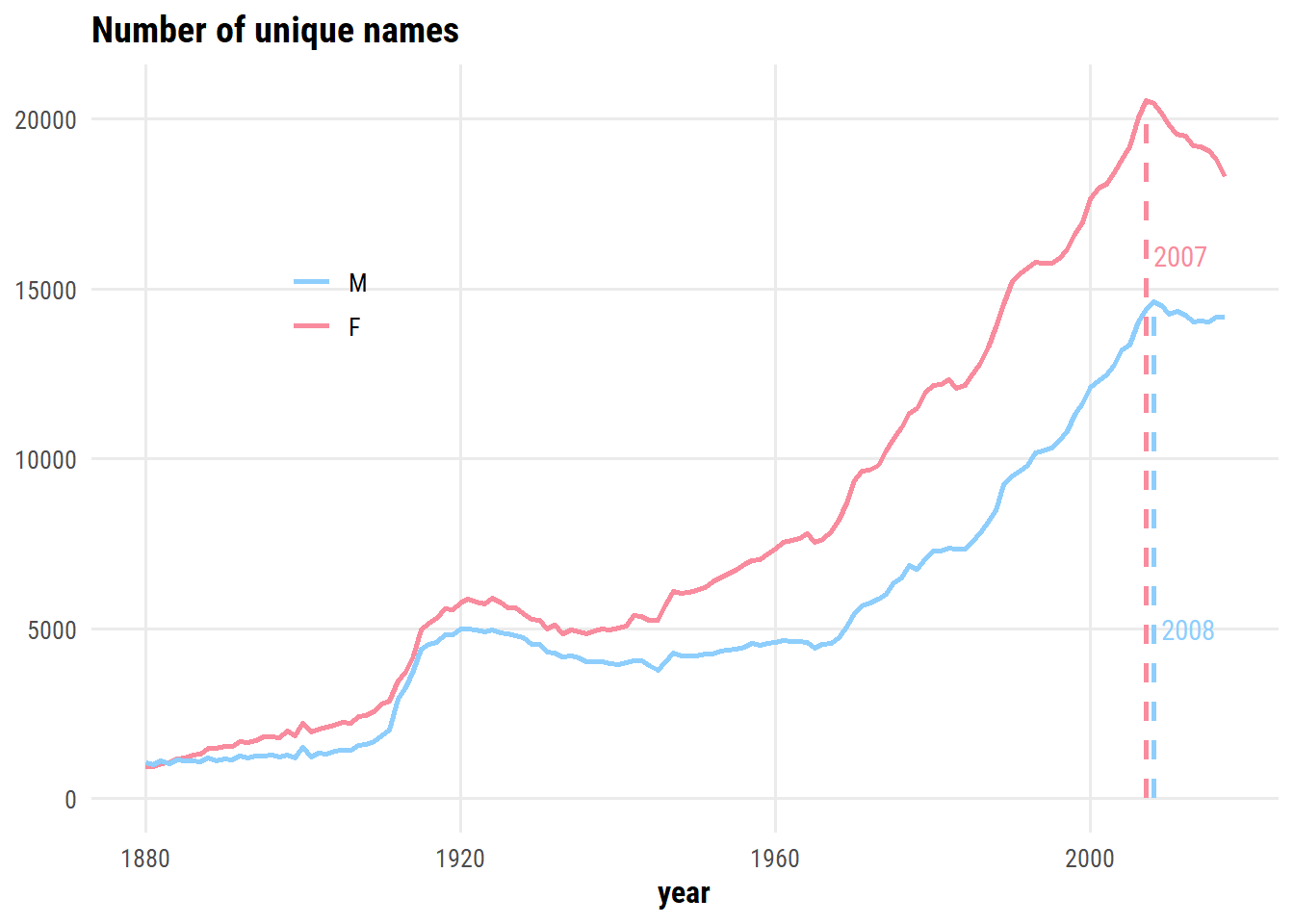There is an dip in the number of unique names after peaking in 2007 and 2008 – I’m assuming this is related to a decrease in overall births following the financial crisis. If we normalize by the number of births, does this trend still appear?

``````d <- babynames %>%
group_by(year, sex) %>%
summarise(n_names = n(), n_births = sum(n),
names_per_1000_births = 1000 * n_names / n_births,
.groups = "drop")
d_max <- d %>%
group_by(sex) %>%
filter(names_per_1000_births == max(names_per_1000_births))

d %>%
ggplot(aes(x = year, y = names_per_1000_births, color = sex)) +
geom_line(size = 1) +
geom_linerange(
data = d_max,
aes(ymin = 0, ymax = names_per_1000_births,
xmin = year, xmax = year, color = sex),
lty = 2, size = 1, show.legend = FALSE
) +
geom_text(
data = d_max,
aes(x = year, y = c(2.5, 3.5), label = year),
hjust = 0, nudge_x = 1, show.legend = FALSE
) +
labs(title = "Number of unique names / 1000 births",
y = NULL, color = NULL) +
scale_color_manual(values = sex_pal) +
theme(legend.position = c(0.5, 0.7))``````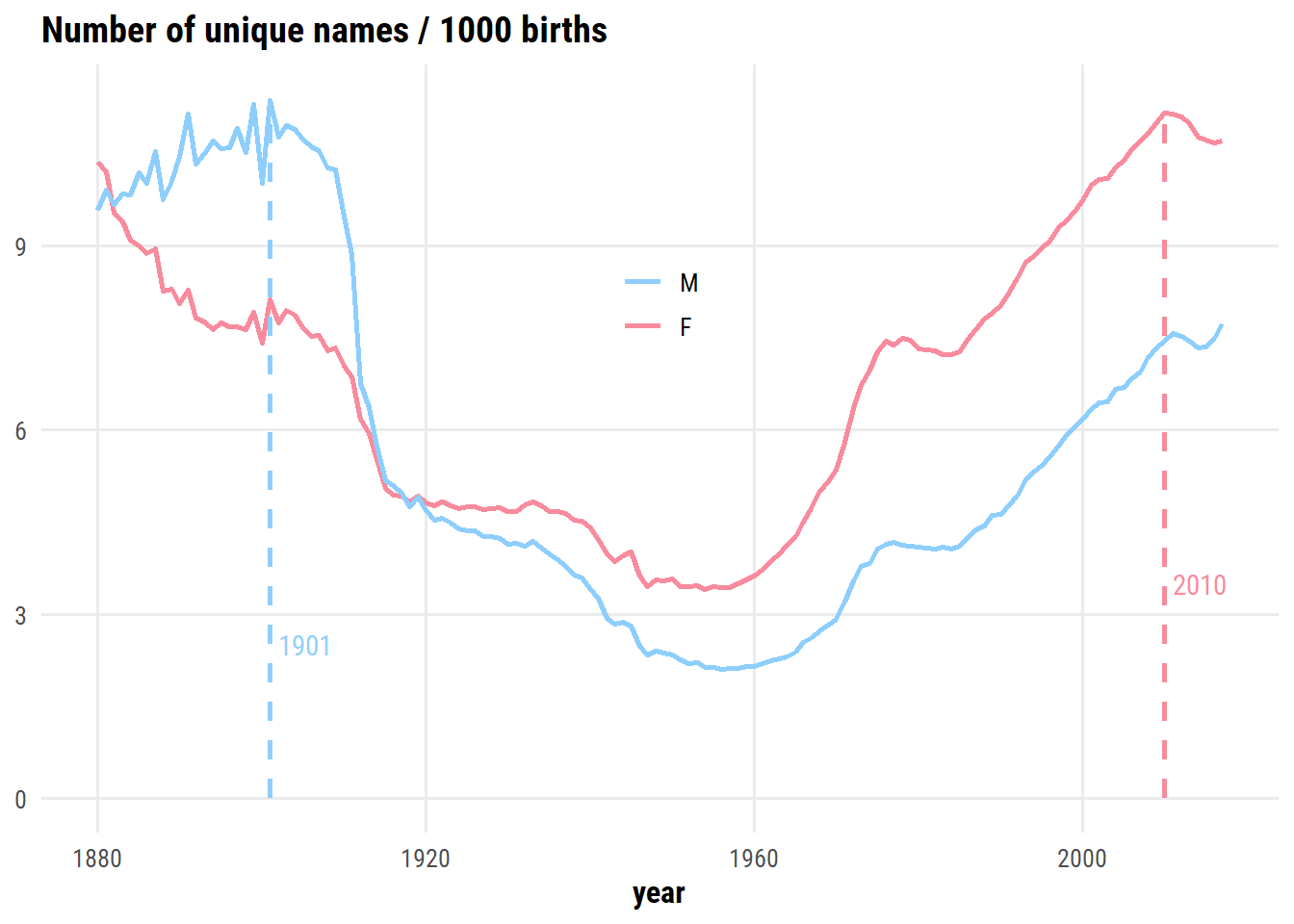Creativity for male names peaked around 1900, and is on the rise again. Creativity for female names has been higher than male names since ~1920, and peaked in 2010.

Another way to think about creativity is the proportion of babies being given the most popular names in a given year:

``````d <- babynames %>%
group_by(year, sex) %>%
mutate(name_rank = rank(-n)) %>%
ungroup() %>%
# Try a few different cut-offs
crossing(top_x = c(10, 25, 50, 100, 200)) %>%
mutate(top_name = name_rank <= top_x)  %>%
group_by(year, sex, top_x) %>%
summarise(p_top = sum(n[top_name]) / sum(n), .groups = "drop")
d %>%
mutate(top_x = fct_reorder(paste0("Top ", top_x, " names"), top_x)) %>%
ggplot(aes(x = year, y = p_top, color = sex)) +
geom_line(size = 1) +
facet_wrap(~ top_x) +
scale_color_manual(values = sex_pal) +
theme(legend.position = c(0.8, 0.2))``````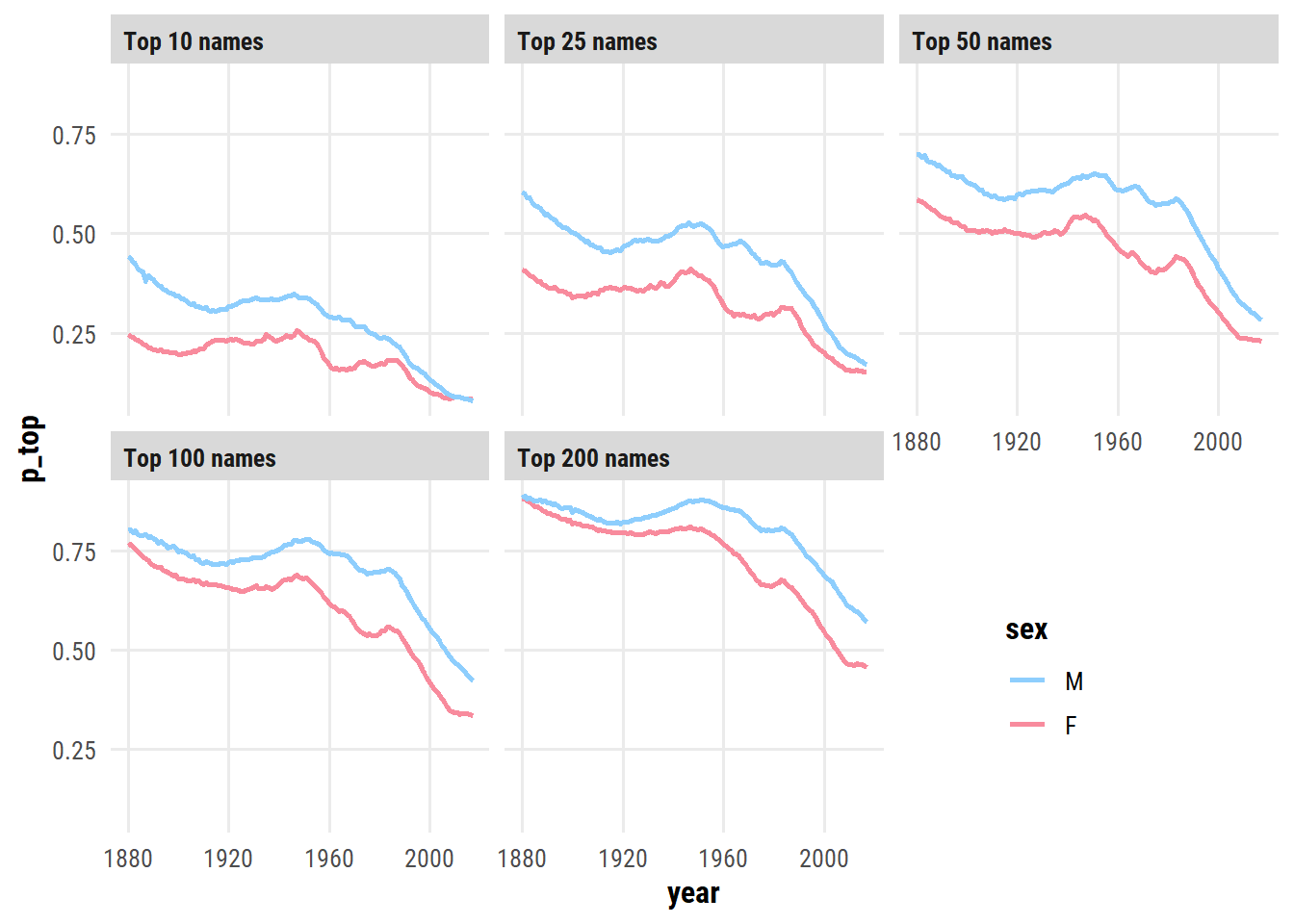Out of these, I prefer the top 50 visualization:

``````d %>%
filter(top_x == 50) %>%
ggplot(aes(x = year, y = p_top, color = sex)) +
geom_line(size = 1) +
scale_y_continuous(NULL, limits = c(0, 1), labels = scales::percent) +
scale_color_manual(values = sex_pal) +
labs(
x = NULL,
title = paste0("Baby <span style='color:#8ecefd'>boy</span> and ",
"<span style='color:#f88b9d'>girl</span> names ",
"are getting more creative over time"),
subtitle = "Proportion of names in the top 50 most common versus year"
) +
theme(legend.position = "none", plot.title = element_markdown())``````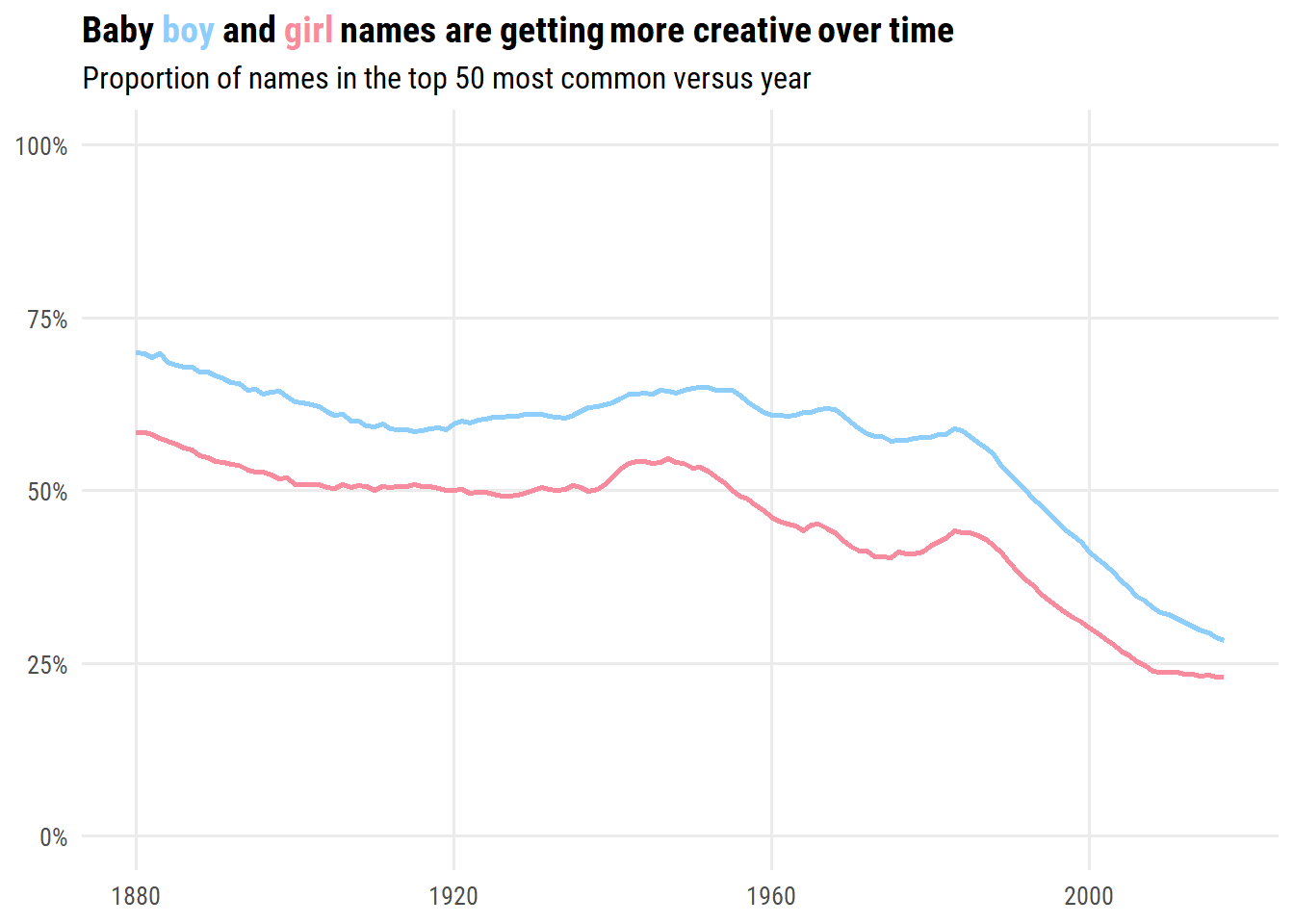## Gender-neutral names

Before getting into gender-neutral names, I’m curious which are the least gender-neutral in this data?

``````d <- babynames %>%
group_by(name, sex) %>%
summarise(n = sum(n), .groups = "drop") %>%
complete(name, sex, fill = list(n = 0)) %>%
pivot_wider(names_from = sex, values_from = n) %>%
rename(female = `F`, male = M) %>%
mutate(total = female + male) %>%
arrange(desc(total)) %>%
filter(female == 0 | male == 0) %>%
select(-total) %>%
pivot_longer(cols = -name, names_to = "sex", values_to = "count") %>%
filter(count > 0) %>%
mutate(name = fct_reorder(name, count))

d %>%
group_by(sex) %>%
slice_max(count, n = 10) %>%
ggplot(aes(y = name, x = count, fill = sex)) +
geom_col() +
facet_wrap(~ sex, nrow = 1, scales = "free_y") +
scale_fill_manual(values = c("#f88b9d", "#8ecefd")) +
labs(
y = NULL, x = NULL,
title = paste0("Top 10 most common ",
"<span style='color:#f88b9d'>100% female</span> and ",
"<span style='color:#8ecefd'>100% male</span> names"),
subtitle = "US baby names from 1880 to 2017"
) +
theme(legend.position = "none", plot.title = element_markdown(),
panel.grid.major.y = element_blank(),
strip.background = element_blank(), strip.text = element_blank())``````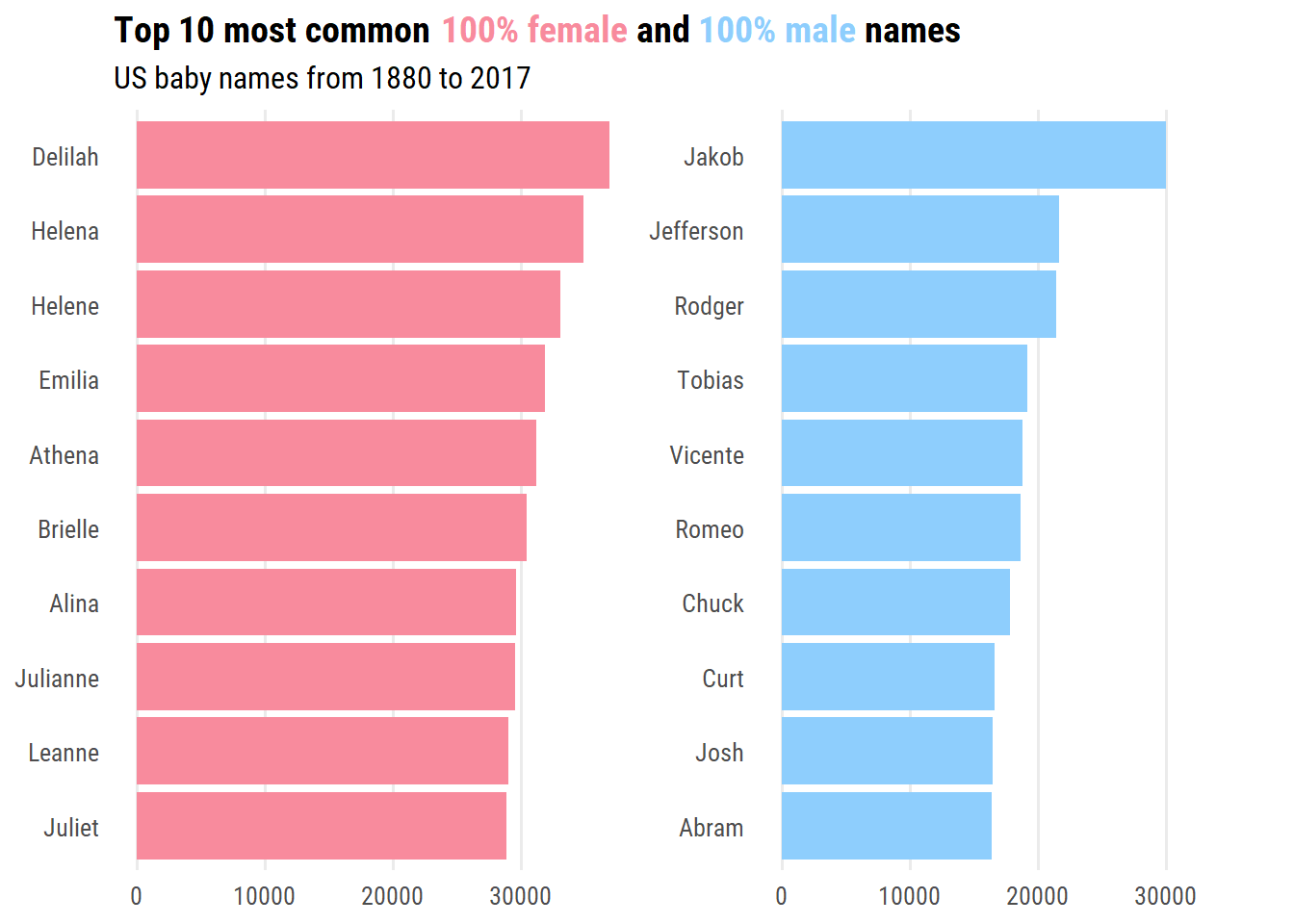Determining the most popular gender-neutral names is not as straightforward. I’ll include names with 40-60% of males/females (over all years), and order by top 10 frequency:

``````d <- babynames %>%
group_by(name) %>%
summarise(n_total = sum(n), p_female = sum(n[sex == "F"]) / n_total)
d %>%
filter(p_female > 0.4, p_female < 0.6) %>%
slice_max(n_total, n = 9) %>%
left_join(babynames, by = "name") %>%
mutate(
name = fct_reorder(name, n_total),
name_label = ifelse(
n_total == max(n_total),
glue("{name} ({scales::percent(p_female, 0.1)} female)"),
glue("{name} ({scales::percent(p_female, 0.1)})")
) %>%
fct_reorder(desc(n_total))
) %>%
ggplot(aes(x = year, y = n)) +
geom_area(aes(fill = sex)) +
facet_wrap(~ name_label, scales = "free_y", nrow = 3) +
scale_fill_manual(values = sex_pal) +
labs(
y = "Births", x = NULL, fill = NULL,
title = "The most frequent gender-neutral names (40-60% male/female)"
) +
theme(legend.position = c(0.1, 0.9))``````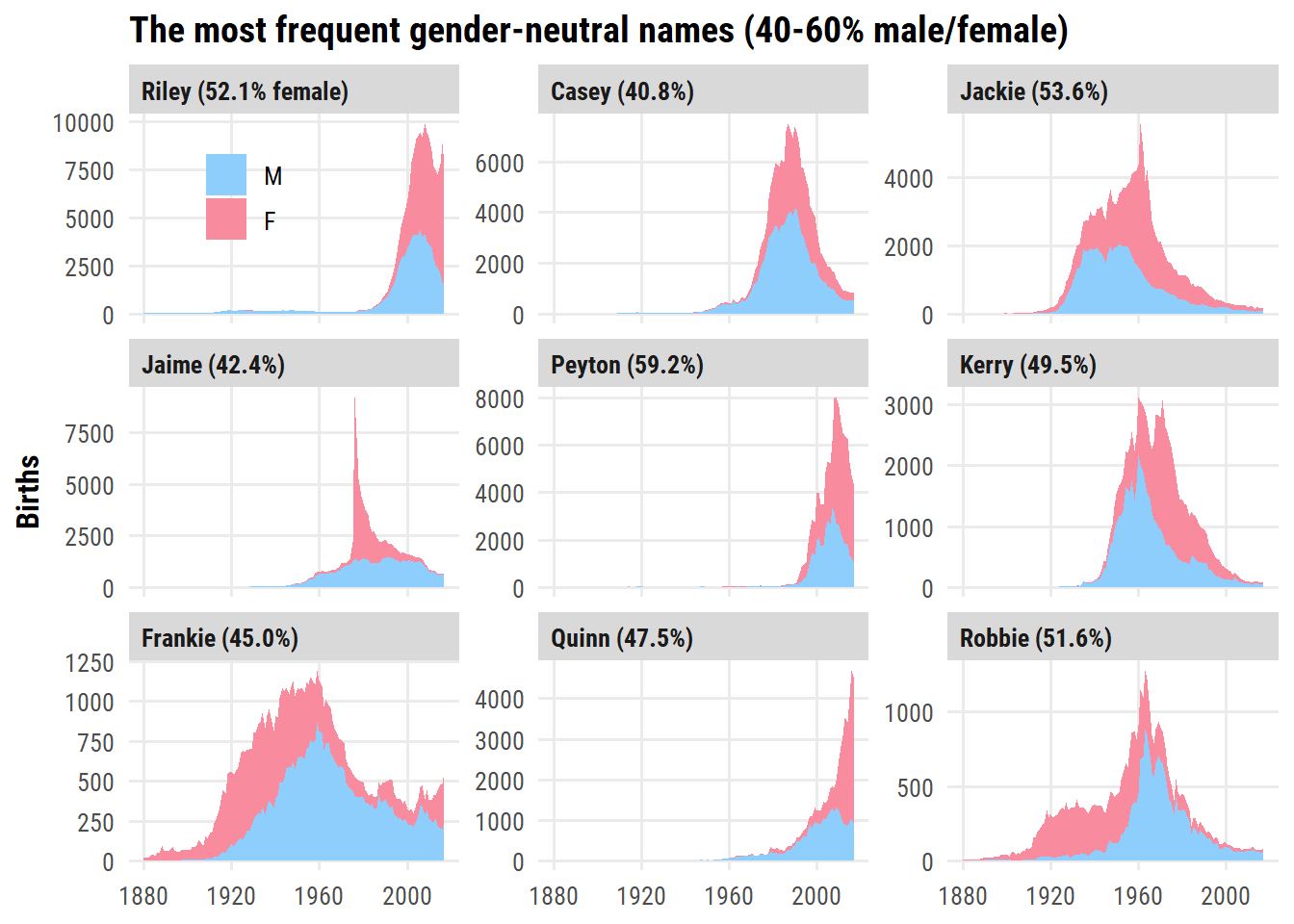Next, I’d like to visualize how the number of gender-neutral names changed over time. To do this, I’ll compute the ratio of females to males for each name in a given year (excluding names which have 0 females or 0 males):

``````d <- babynames %>%
group_by(year, name) %>%
# Keep only names with both sexes
filter("F" %in% sex, "M" %in% sex) %>%
summarise(ratio_female = n[sex == "F"] / n[sex == "M"], .groups = "drop")
glimpse(d)``````
``````Rows: 168,381
Columns: 3
\$ year         <dbl> 1880, 1880, 1880, 1880, 1880, 1880, 1880, 1880, 1880, 188…
\$ name         <chr> "Addie", "Allie", "Alma", "Alpha", "Alva", "Anna", "Annie…
\$ ratio_female <dbl> 3.425000e+01, 3.387097e+00, 1.978571e+01, 4.333333e+00, 2…``````

Then I’ll plot each ratio as a point and use the `ggpointdensity` package to color by the density of points:

``````d %>%
ggplot(aes(x = year, y = ratio_female)) +
ggpointdensity::geom_pointdensity(size = 3, adjust = 1, alpha = 0.2,
show.legend = FALSE) +
dunnr::scale_color_td(palette = "div5", type = "continuous") +
scale_y_log10(breaks = 10^seq(-2, 2),
labels = c("1:100", "1:10", "1:1", "10:1", "100:1")) +
labs(
x = NULL, y = NULL,
title = "The amount of gender-neutral names is increasing",
subtitle = "Each point represents the ratio of females:males for a single name in a single year.\nColor indicates the density of points.",
caption = paste("data: {babynames} R package",
"plot: Taylor Dunn", sep =  " | ")
) +
theme(plot.subtitle = element_text(size = 10))``````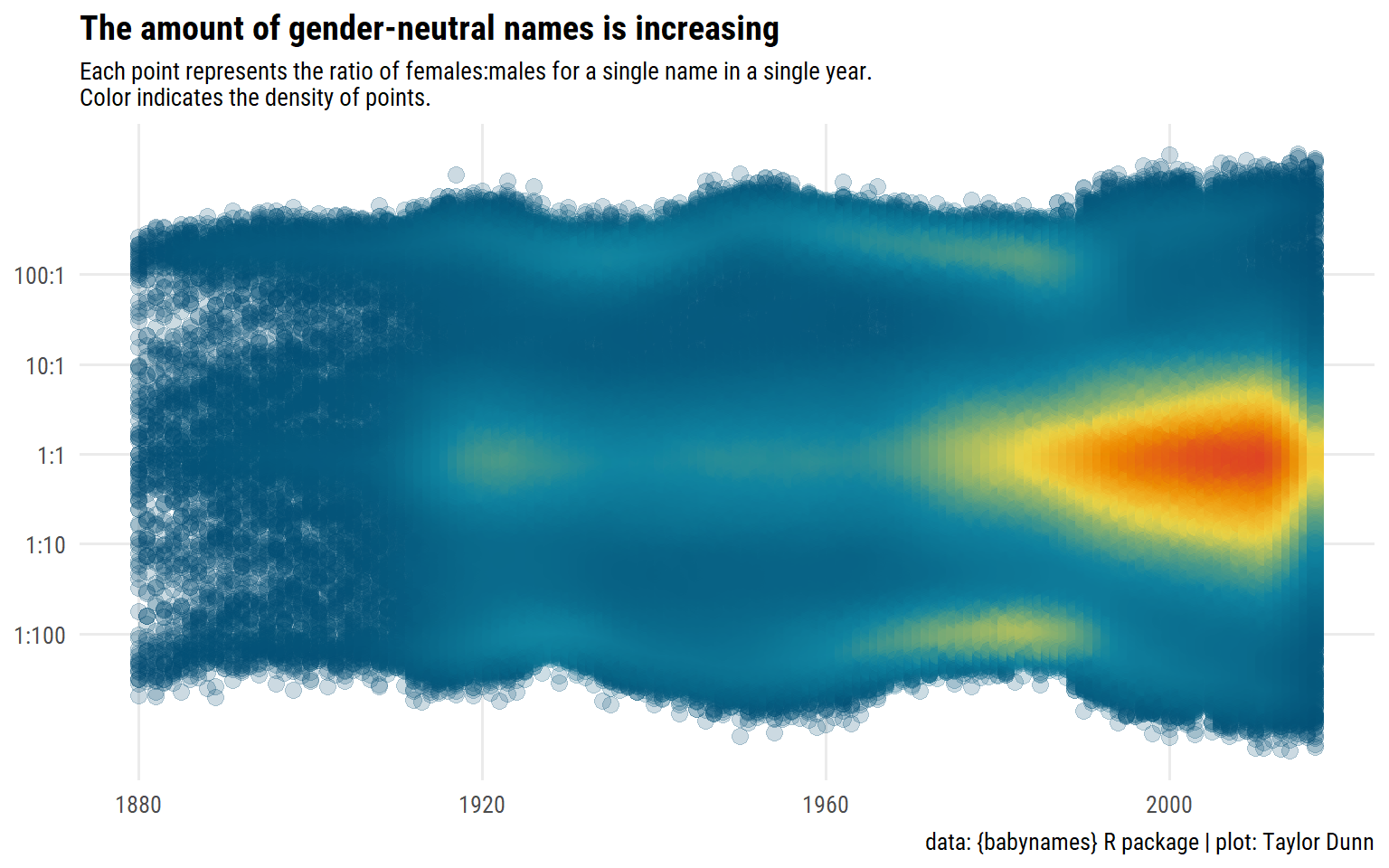## My name

Selfishly, I of course want to explore my name and its different variations.

First, here are the names which start with “Tayl” or “Tail” and have an “r” in them:

``````babynames_taylor <- babynames %>%
filter(str_detect(name, "Tayl|Tail") & str_detect(name, "r")) %>%
group_by(name) %>%
mutate(n_total = sum(n)) %>%
ungroup()
babynames_taylor %>%
distinct(name, n_total) %>%
arrange(desc(n_total)) %>%
rmarkdown::paged_table()``````

Unsurprised to see “Taylor” as the most common spelling. I can’t say I’ve met anyone with a double-name like “Taylorann” or “Taylormarie” before.

I’ll take the top 6 from these and plot births over time:

``````babynames_taylor %>%
distinct(name, n_total) %>%
slice_max(n_total, n = 6) %>%
left_join(babynames_taylor %>% select(-n_total), by = "name") %>%
group_by(name, sex) %>%
mutate(n_cumsum = cumsum(n)) %>%
ungroup() %>%
mutate(
name_label = glue("{name} (n = {n_total})") %>% fct_reorder(desc(n_total))
) %>%
ggplot(aes(x = year, y = n, fill = sex)) +
geom_area() +
scale_fill_manual(values = sex_pal) +
facet_wrap(~ name_label, scales = "free_y") +
coord_cartesian(xlim = c(1960, 2020)) +
labs(y = "Births", x = "Year", fill = NULL,
title = "Top 6 variations of 'Taylor'") +
theme(legend.position = c(0.1, 0.9))``````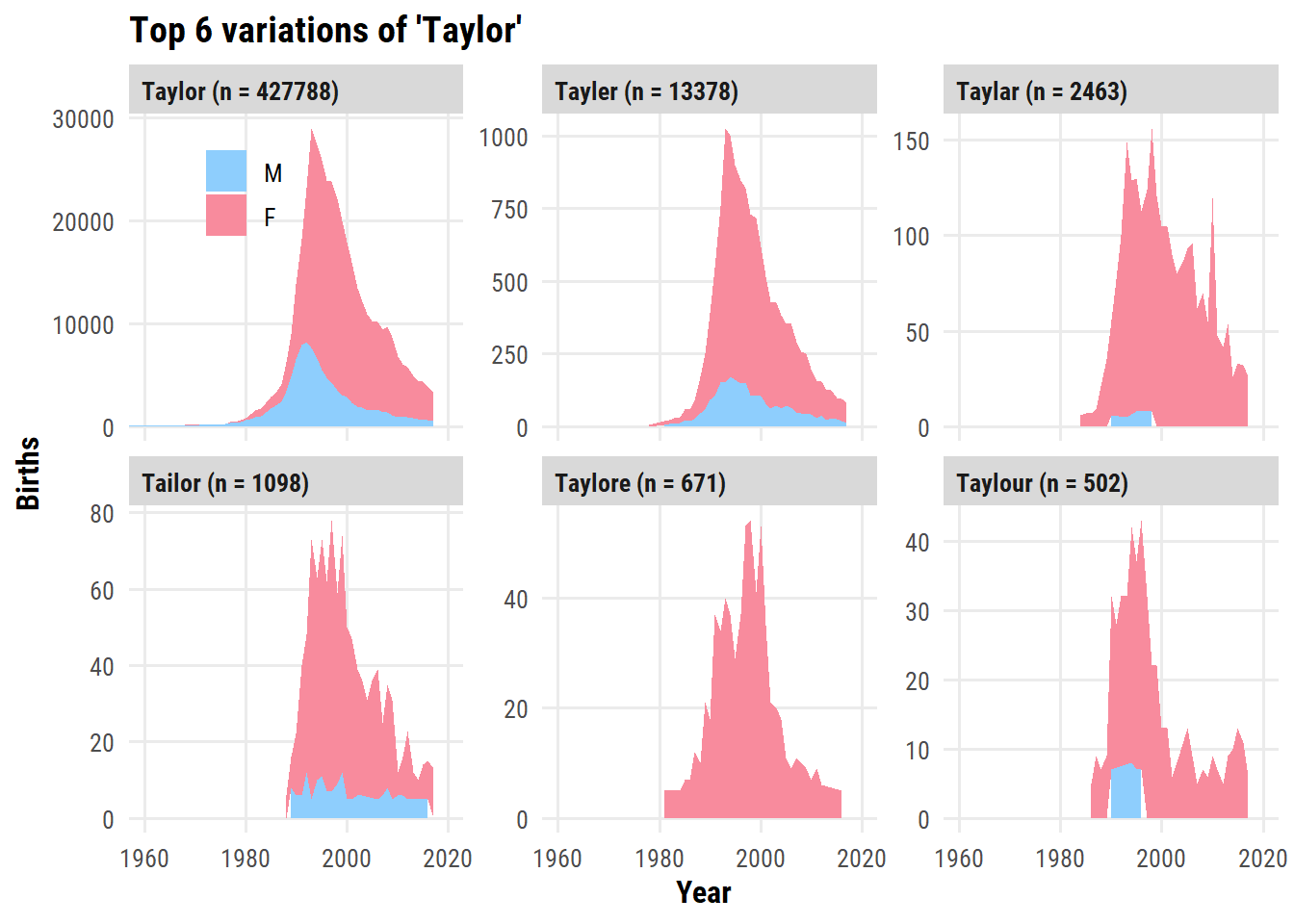Now just focusing on “Taylor”, which year was the name most popular for each sex?

``````babynames_taylor %>%
group_by(sex) %>%
filter(n == max(n)) %>%
ungroup() %>%
select(sex, year, n) %>%
gt()``````
sex year n
M 1992 8240
F 1993 21266

The most popular year for male Taylors happens to be the year I was born: 1992. Female Taylors peaked in frequency the next year.

Lastly, a plot of the proportion and number of Taylors by sex over time:

``````d <- babynames_taylor %>%
filter(name == "Taylor", year >= 1960) %>%
select(year, sex, n) %>%
complete(year, sex, fill = list(n = 0)) %>%
group_by(year) %>%
mutate(p_female = n[sex == "F"] / sum(n)) %>%
ungroup()
p_title <- glue(
"<span style='color:{sex_pal}'>Male</span> and ",
"<span style='color:{sex_pal}'>Female</span> Taylors over time",
)
p1 <- d %>%
distinct(year, p_female) %>%
ggplot(aes(x = year)) +
geom_ribbon(aes(ymin = 0, ymax = p_female), fill = sex_pal["F"]) +
geom_ribbon(aes(ymin = p_female, ymax = 1.0), fill = sex_pal["M"]) +
geom_line(aes(y = p_female), color = "white", size = 1) +
geom_hline(yintercept = 0.5, lty = 2, color = "white", size = 1) +
scale_y_continuous(labels = scales::percent,
expand = c(0, 0)) +
scale_x_continuous(breaks = NULL, expand = c(0, 0)) +
labs(x = NULL, y = "Proportion by sex", title = p_title) +
theme(plot.title = element_markdown())
p2 <- d %>%
ggplot(aes(x = year, y = n, fill = sex)) +
geom_area() +
scale_fill_manual(values = sex_pal) +
scale_x_continuous("Year", breaks = seq(1960, 2020, 10), expand = c(0, 0)) +
theme(legend.position = "none", panel.grid.major.x = element_blank()) +
labs(y = "Number of births")

p1 / p2``````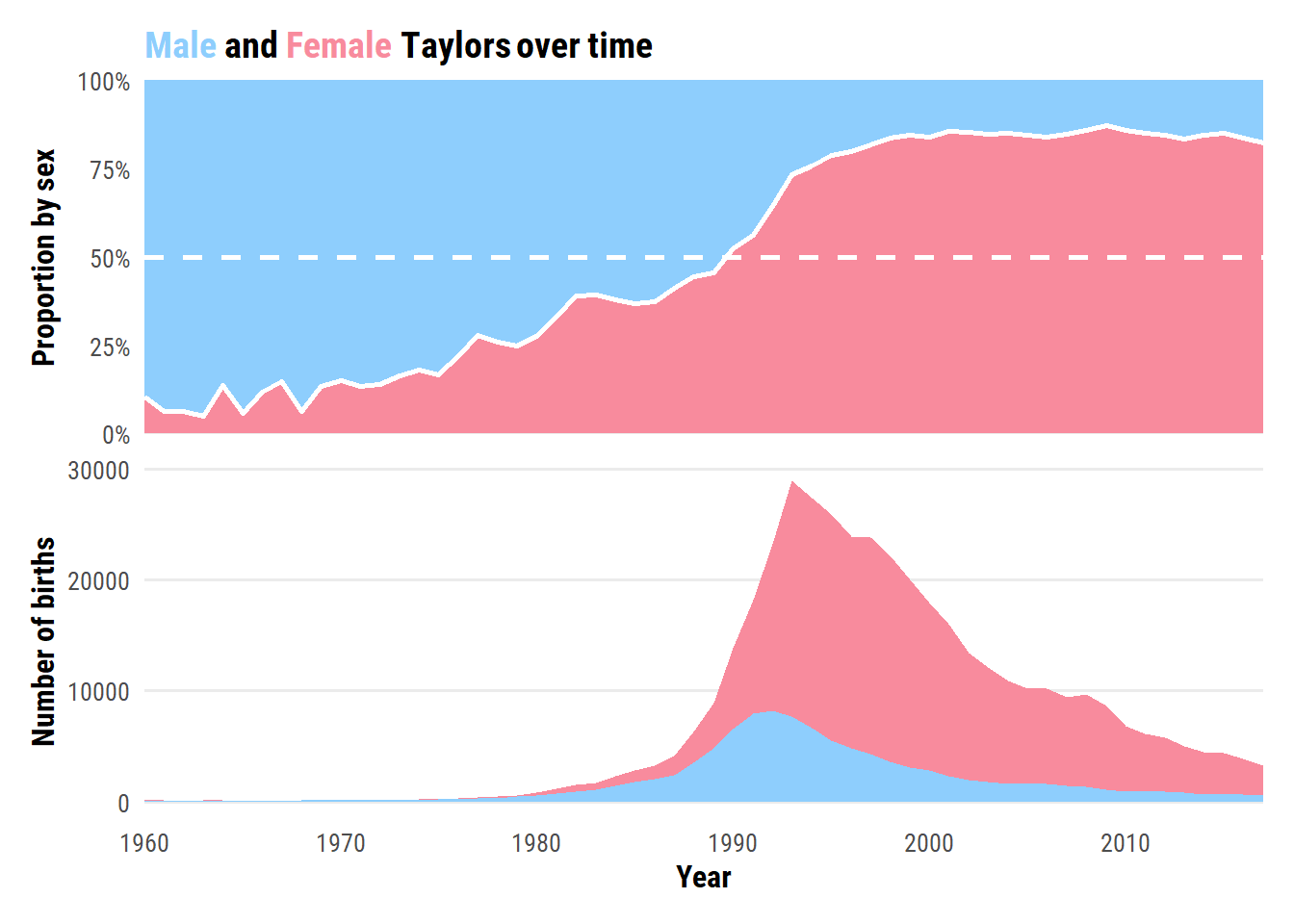## Reproducibility

Session info
`````` setting  value
version  R version 4.2.1 (2022-06-23 ucrt)
os       Windows 10 x64 (build 19044)
system   x86_64, mingw32
ui       RTerm
language (EN)
tz       America/Curacao
date     2022-10-27
pandoc   2.18 @ C:/Program Files/RStudio/bin/quarto/bin/tools/ (via rmarkdown)``````
Git repository
``````Local:    main C:/Users/tdunn/Documents/tdunn-quarto
Remote:   main @ origin (https://github.com/taylordunn/tdunn-quarto.git)

## Citation

BibTeX citation:
``````@online{dunn2022,
author = {Dunn, Taylor},
title = {Analyzing {US} Baby Names over the Years},
date = {2022-03-26},
url = {https://tdunn.ca/posts/2022-03-26-tidytuesday-2022-week-12},
langid = {en}
}
``````